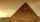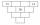# Pyramid + reason - math problems

#### Number of problems found: 5

• TowerCharles built a tower of cubes with an edge 2 cm long. In the lowest layer there were 6 cubes (in one row) in six rows, in each subsequent layer always 1 cube and one row less. What volume in cm3 did the whole tower have?
• Bricks pyramidHow many 50cm x 32cm x 30cm brick needed to built a 272m x 272m x 278m pyramid?
• Pyramid Z8–I–6Each brick of the pyramid contains one number. Whenever possible, the number in each brick is the lowest common multiple of two numbers of bricks lying directly above it. May that number be in the lowest brick? Determine all possibilities.
• Billiard ballsA layer of ivory billiard balls of radius 6.35 cm is in the form of a square. The balls are arranged so that each ball is tangent to every one adjacent to it. In the spaces between sets of 4 adjacent balls other balls rest, equal in size to the original.
• Hexagon rotationA regular hexagon of side 6 cm is rotated through 60° along a line passing through its longest diagonal. What is the volume of the figure thus generated?

We apologize, but in this category are not a lot of examples.
Do you have an exciting math question or word problem that you can't solve? Ask a question or post a math problem, and we can try to solve it.

We will send a solution to your e-mail address. Solved examples are also published here. Please enter the e-mail correctly and check whether you don't have a full mailbox.

Pyramid Problems. Reason - math problems.